## 理解CSS3 transform中的Matrix(矩阵)

### 一、哥，我被你吓住了

CSS同行间：

CSS门槛低，无需程序基础或数学逻辑能力，也能做出点自我感觉不错的东西。然而，你自己也应该清楚的，一般你能轻松学到的东西，别人也可以。因此，如果你想挤进那20%的行列，就要学到一般人学不到的深度，学到一般人学不了的东西。自然，是需要更多额外的努力的。如果每次你都比别人努力一点点，何愁不比他人高出几等。人年轻的时候，贵在坚持！

### 二、何为矩阵？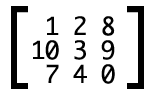CSS3中的矩阵
CSS3中的矩阵指的是一个方法，书写为`matrix()``matrix3d()`，前者是元素2D平面的移动变换(transform)，后者则是3D变换。2D变换矩阵为3*3, 如上面矩阵示意图；3D变换则是4*4的矩阵。

```.trans_skew { transform: skew(35deg); }
.trans_scale { transform:scale(1, 0.5); }
.trans_rotate { transform:rotate(45deg); }
.trans_translate { transform:translate(10px, 20px); }
```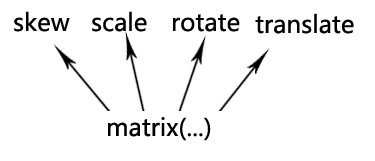OK，现在上面提到的CSS3矩阵解释应该说得通了。

### 四、`transform`与坐标系统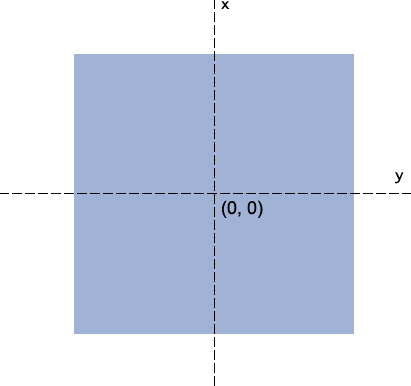`-webkit-transform-origin: bottom left;``transform-origin: 50px 70px;`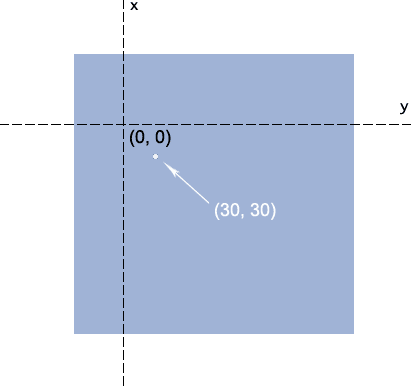### 五、准备好了没？重头戏来了

CSS3 `transform``matrix()`方法写法如下：

`transform: matrix(a,b,c,d,e,f);`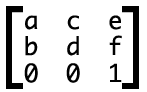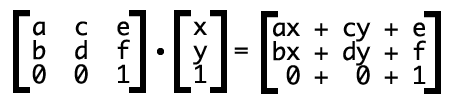//zxx:大学时候线性代数知识，懂的人这里可以直接跳过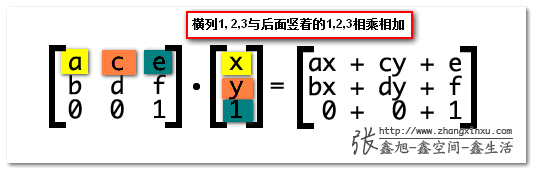`ax+cy+e`的意义是什么？

`transform: matrix(1, 0, 0, 1, 30, 30); /* a=1, b=0, c=0, d=1, e=30, f=30 */`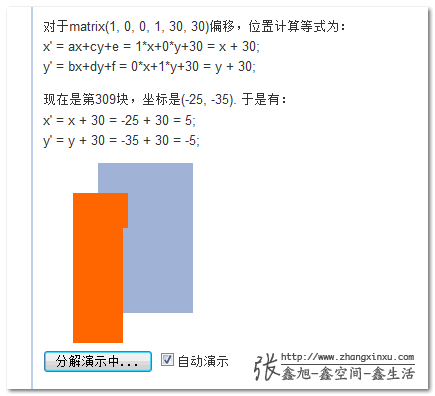//zxx:由于默认100毫秒间隔不断渲染，因此如果你电脑CPU或是浏览器hold不住，可以取消“自动演示”的勾选，然后，点击左边的按钮手动分步查看。

`transform: matrix(与我无关, 哪位, 怎么不去高考, 打麻将去吧, 水平偏移距离, 垂直偏移距离);`

### 六、transform matrix矩阵与缩放，旋转以及拉伸

`transform: matrix(1, 0, 0, 1, 30, 30);`

`x' = ax+cy+e = s*x+0*y+0 = s*x;`
`y' = bx+dy+f = 0*x+s*y+0 = s*y;`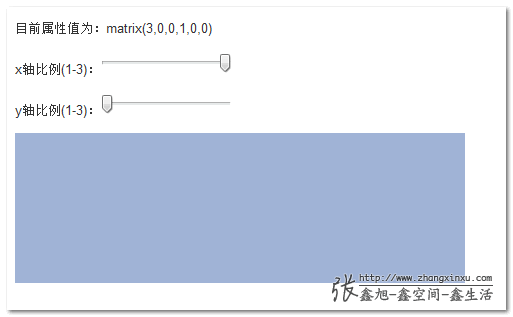`matrix(cosθ,sinθ,-sinθ,cosθ,0,0)`

```x' = x*cosθ-y*sinθ+0 = x*cosθ-y*sinθ
y' = x*sinθ+y*cosθ+0 = x*sinθ+y*cosθ```

`filter:progid:DXImageTransform.Microsoft.Matrix(M11=cosθ,M21=sinθ,M12=-sinθ,M22=cosθ');`

CS-SC：初三-上床，对称结构，这下忘不了了吧~~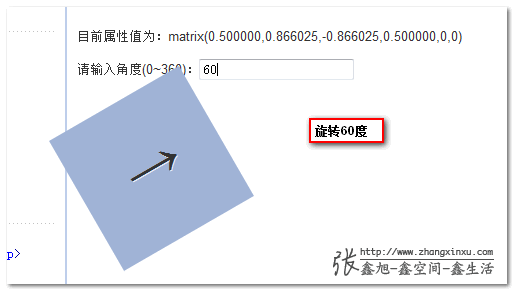`transform:rotate(30deg);`

`transform: matrix(0.866025,0.500000,-0.500000,0.866025,0,0);`

`matrix(1,tan(θy),tan(θx),1,0,0)`

```x' = x+y*tan(θx)+0 = x+y*tan(θx)
y' = x*tan(θy)+y+0 = x*tan(θy)+y```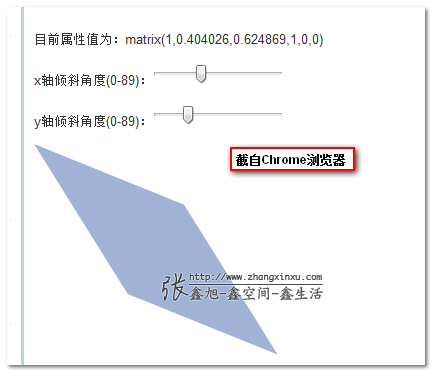### 七、既然有简单的skew, rotate..，那matrix有何用？

OK，这里就演示下，如何使用CSS3 `transform` `matrix`矩阵实现镜像效果。demo页面中的一个轴是为了便于理解我加上的效果，实际上，在镜像对称的时候轴是看不见的。

`matrix((1-k*k) / (1+k*k), 2k / (1 + k*k), 2k / (1 + k*k), (k*k - 1) / (1+k*k), 0, 0)`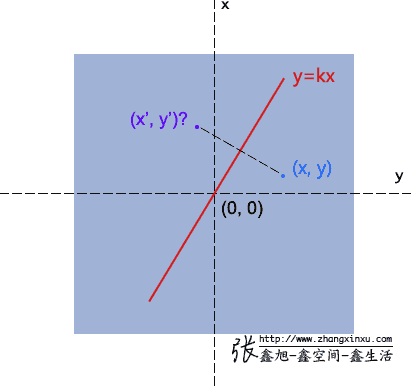`(y-y') / (x - x') = -1/ k → ky-ky' = -x+x'`
`(x + x') / 2 * k = (y + y')/2 → kx+kx' = y+y'`

`x' = (1-k*k)/(k*k+1) *x + 2k/(k*k+1) *y;`
`y' = 2k/(k*k+1) *x + (k*k-1)/(k*k+1) *y;`

`x' = ax+cy+e;`
`y' = bx+dy+f;`

`a = (1-k*k)/(k*k+1);`
`b = 2k/(k*k+1);`
`c = 2k/(k*k+1);`
`d = (k*k-1)/(k*k+1);`### 八、3D变换中的矩阵

3D变换虽然只比2D多了一个D，但是复杂程度不只多了一个。从二维到三维，是从4到9；而在矩阵里头是从3*3变成4*4, 9到16了。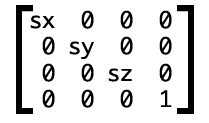`transform: matrix3d(sx, 0, 0, 0, 0, sy, 0, 0, 0, 0, sz, 0, 0, 0, 0, 1)`

### 九、结束语

（本篇完）

﻿
1. 解决方案说道：

如何实现垂直翻转

2. 小天神功说道：

我记得好像有本教材选修叫做《矩阵与变换》，要不作者讲讲吧，我觉得按那种方式我觉得可能更好一些，我现在暂时没有资金去买，我明年尽量去网上淘一本

3. 我的眼镜就是尺说道：

例子中的对称点可以用这个
transform: rotateZ(90deg) rotateY(180deg);
或这个transform: rotate3d(-1, 1, 0, 180deg);

4. 胡小小说道：

厉害,受教了

5. Logan说道：

太牛了

6. 3d矩阵说道：

老哥你这sD矩阵的东西怎末这么少，还行看你在解释一下呢，就只有个缩放，再搞几个呀

7. warma说道：

nb

8. saifjhis说道：

我真的佛了，做一个圆根据百分比展现部分内容的饼图，但用CSS跟屎一样，好不容易画出来了， 又因为这个transform角度一直调不来，我还是回归canvas把，太痛苦了！！！

• 小龙说道：

9. Johnson说道：

“但是，矩阵计算的原来是一致的。”，似乎有个错别字，”原来“应该是”原理“吧？

10. Logic说道：

卧槽我理解不了，我的智商，啊，脑壳痛，卧槽…

• 说道：

我现在也是这个状态…

11. js heap过高说道：

老哥，你这个页面js heap持续变高啊，检查下吧

12. 18814121923@163.com说道：

大佬，矩阵[a c e b d b f 0 0 1]为什么最后一行是[0 0 1]不是[0 0 0]

• hateko说道：

001是补位，前六个参已经描述出来图形变换对应的参数了，但是不是一个3*3的矩阵，后续还要跟锚点坐标计算所以补位成3*3，000的话有的参数就算没了，就是线性代数

旋转那个是不是有点问题，点（0，6）旋转30°，按照公式算那就是（-3，3根号3），但实际应该是（3，3根号3）

14. 艾琳说道：

楼主，我遇到一个问题，当matrix的xx,yy一定大的时候，浏览器就没有transition动画了，而是先消失再可见，这是为何？

15. Li先生说道：

transform: matrix(0.866025,0.500000,-0.500000,0.866025,0,0);这六个数值都代表了什么？请问大佬能帮忙解答一下吗。

matrix（cos30°,sin30°,-sin30°,cos30°,0,0)

• API说道：

matrix(scaleX(),skewY(),skewX(),scaleY(),translateX(),translateY())

16. Megamouth说道：

2D矩阵中第三行参数 001，和坐标中第三行参数 1 的意义是什么？

17. demo说道：

事实上56收14影响

18. geylnu说道：

因为touch事件没有offsetX Y属性，简单模拟的对transform元素有异常，后来根据getComputedStyle获取的transform属性查到这里来了，原来变换都是通过矩阵完成的，完美解决！

19. CC娇说道：

“然而，这却是屌丝逆袭的一个好机会。”
就喜欢这种感觉！

20. febody说道：

有一个问题啊，请教下大侠

用matrix 矩阵表示旋转，如果我直接写 transform: rotate(360deg),在加上animation 是可以有动画效果的

但是如果直接用matrix表示，360deg和0deg 算出来的东西是一样的，那么相当于矩阵前后一样，不会有动画，也就是说matrix 表示整圈数 的时候n*360deg的时候，都是一样的，不会触发animation，，这个是不是很尴尬啊，想半天没啥好的解决办法，请问你有过这方面考虑吗

21. 苏哇说道：

为什么 matrix与镜像对称效果demo 例子中 ，这里需要用负数？
比如旋转60度，使用rotate会顺时针，但一般直角坐标系是逆时针旋转的原因？

// 确认对称线
var k = Math.tan(-1 * value * Math.PI / 180)

22. 李运华说道：

看了这篇文章 我把作者的书买了。。虽然是搞后台的。

23. 天籽聪慧说道：

说说我的一些对于matrix的理解，我也遇到同时缩放和旋转的问题，用matrix的话，(a,b,c,d,e,f)主要是缩放的时候不单是a和d的缩放，同时bc也需要，假如放大两倍的话，abcd都要同时放大两倍。举个简单的例子，一个图形放大两倍并顺时针旋转三十度的话，先旋转(0.866025,0.500000,-0.500000,0.866025,0,0)然后乘2，得到(1.73205,1,-1,1.73205,0,0)，这就是同时缩放和旋转的问题

• delen说道：

b,c两个值改变与否不会影响缩放

24. 匆匆的来说道：

哥，你的XY轴画反了

• 小也想吃粉说道：

是的…俺也发现了

25. Sghen说道：

已知matrix，如何求translate scale skew和rotate

26. zihan说道：

transform: matrix(与我无关, 哪位, 怎么不去高考, 打麻将去吧, 水平偏移距离, 垂直偏移距离);

水平偏移距离, 垂直偏移距离 如果值是百分比怎么办?

• Li先生说道：

水平偏移距离, 垂直偏移距离 怎么获取呢

27. 游客说道：

给大佬点赞

28. naice说道：

我同时要旋转位移缩放。那怎么搞？？

• bigHorse说道：

你可能需要hammer.js去寻找一些思路

• 游客说道：

也许，线性代数的矩阵变换欢迎你？

• loro说道：

transform:matrix(0.866025,0.500000,-0.500000,0.866025,0,0) matrix(3, 0, 0, 2, 0, 0)；用空格隔开

29. 小咸鱼说道：

大佬，这态度，这解释，深入浅出，大佬大佬

30. 懵懂小书童说道：

必须为真诚的态度点不赞

31. k644说道：

得补下数学知识，有些公式实在看不懂，暂时只能死记

32. haley说道：

一直在关注鑫哥的博客，受益匪浅

33. hey说道：

所以这就是没有办法算出元素旋转超过360度的angle值的原因吗

34. 觉得对称点的计算有问题说道：

读了一篇2d的觉得元气大伤,可能需要回去补个血才能继续看3d的

35. 觉得对称点的计算有问题说道：

觉得对称点的计算有问题,
y= kx
y’ = kx’

那么应该有( y’ – y) = k(x’ – x) ,那么有(y-y’) / (x – x’) = k ,我不明白为什么你会等于 – 1/k.
求解,我没有推导出来这个结果.
x’ = (1-k*k)/(k*k+1) *x + 2k/(k*k+1) *y;
y’ = 2k/(k*k+1) *x + (k*k-1)/(k*k+1) *y;

• 对称点没啥问题说道：

两条直线垂直,它们的斜率互为负倒数，高中的数学

36. 板栗17说道：

想不通k的取值为啥不是 Math.tan(45)。 而是var k = Math.tan( -1 * value * Math.PI / 180),这个，请大神解释一下，谢谢

• 板栗17说道：

哦，知道了，应该是45°，要用π转换一下。

37. ft说道：

transform: matrix(a,b,c,d,e,f); 放在矩阵里面为什么第三行 为0 0 1 ？
还有乘上的单列矩阵 第三行为什么是 1 ？

如果变3d 或更高维度 怎么对应到矩阵去？

• MC说道：

我觉得不一定是1,1是代表了一个点，可能是2,3,4….一直到第n个点，算完了就结束